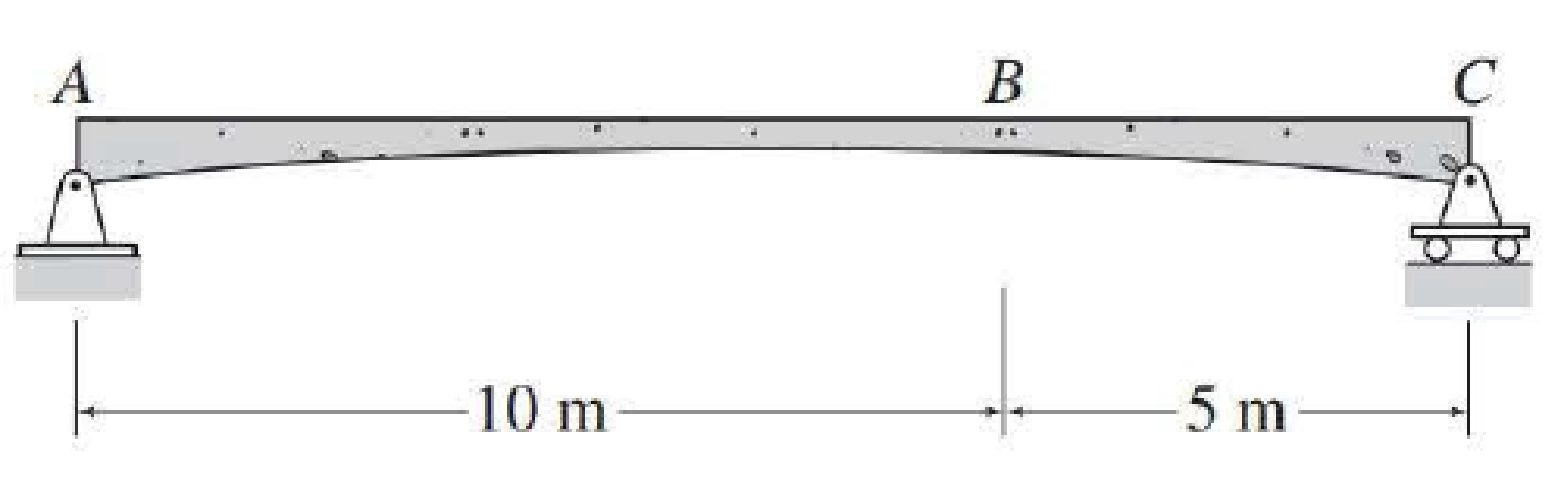# For the beam of Problem 8.3, determine the maximum positive shear and bending moment at point B due to the series of three moving concentrated loads shown in Fig. P9.13. FIG. P9.13, P9.17, P9.18, P9.22 FIG. P8.3

#### Solutions

Chapter
Section
Chapter 9, Problem 13P
Textbook Problem
54 views

## For the beam of Problem 8.3, determine the maximum positive shear and bending moment at point B due to the series of three moving concentrated loads shown in Fig. P9.13.FIG. P9.13, P9.17, P9.18, P9.22FIG. P8.3

To determine

Find the maximum positive shear and bending moment at point B.

### Explanation of Solution

Calculation:

Apply a 1 kN unit moving load at a distance of x from left end A.

Sketch the free body diagram of beam as shown in Figure 1.

Refer Figure 1.

Find the equation of support reaction (Ay) at A using equilibrium equation:

Consider moment equilibrium at point C.

Consider clockwise moment as positive and anticlockwise moment as negative.

Sum of moment at point C is zero.

ΣMC=0Ay(15)1(15x)=0Ay(15)15+x=015Ay=15x

Ay=1x15        (1)

Find the equation of support reaction (By) at B using equilibrium equation:

Apply vertical equilibrium equation of forces.

Consider upward force as positive (+) and downward force as negative ().

Ay+Cy=1

Substitute 1x15 for Ay.

1x15+Cy=1Cy=11+x15Cy=x15        (2)

Influence line for shear at point B.

Find the equation of shear force at B of portion AB (0x<10m).

Sketch the free body diagram of the section AB as shown in Figure 2.

Refer Figure 2.

Apply equilibrium equation of forces.

Consider upward force as positive (+) and downward force as negative ().

ΣFy=0AySB1=0SB=Ay1

Substitute 1x15 for Ay.

SB=1x151=x15

Find the equation of shear force at B of portion BC (10m<x15m).

Sketch the free body diagram of the section BC as shown in Figure 3.

Refer Figure 3.

Apply equilibrium equation of forces.

Consider upward force as positive (+) and downward force as negative ().

ΣFy=0

SB1+Cy=0SB=1Cy

Substitute x15 for Cy.

SB=1x15

Thus, the equations of the influence line for SB are,

SB=x15 0x<10m        (3)

SB=1x15 10m<x15m        (4)

Find the value of influence line ordinate of shear force at various points of x using the Equations (3) and (4) and summarize the value as in Table 1.

 x (m) SB (kN/kN) 0 0 10− −23 10+ 13 16 0

Draw the influence lines for the shear force at point B using Table 1 as shown in Figure 4.

Refer Figure 4.

Find the slope (θAB) of influence line diagram of shear at B in the portion AB.

θAB=23LAB

Here, LAB is the length of the beam from A to B.

Substitute 10 m for LAB.

θAB=2310=115

Find the slope (θBC) of influence line diagram of shear at B in the portion BC.

θBC=13LBC

Here, LBC is the length of the beam from B to C.

Substitute 5 m for LBC.

θBC=135=115

Find the maximum positive shear force at B.

Sketch the loading position on the beam when the load 1 placed at just right of B as shown in Figure 6.

Refer Figure 6.

Find the shear force at B when the load 1 placed at just right of B.

(SB)1=125(LBC)(θBC)+100(LBC2)(θBC)+50(0)

Substitute 5 m for LBC and 115 for θBC.

(SB)1=125(5)(115)+100(52)(115)+50(0)=41.67+20+0=61.67kN

Sketch the loading position on the beam when the load 2 placed at just right of B as shown in Figure 7.

Refer Figure 7.

Find the shear force at B when the load 2 placed at just right of B.

(SB)2=125(LAB2)(θAB)+100(LBC)(θBC)+50(LBC3)(θBC)

Substitute 10 m for LAB, 115 for θAB, 5 m for LBC, and 115 for θBC.

(SB)2=125(102)(115)+100(5)(115)+50(53)(115)=66.67+33.33+6.67=26.67k

Sketch the loading position on the beam when the load 3 placed at just right of B as shown in Figure 8.

Refer Figure 8.

Find the shear force at B when the load 3 placed at just right of B.

(SB)3=125(LAB5)(θAB)+100(LAB3)(θAB)+50(LBC)(θBC)

Substitute 10 m for LAB, 115 for θAB, 5 m for LBC, and 115 for θBC

### Still sussing out bartleby?

Check out a sample textbook solution.

See a sample solution

#### The Solution to Your Study Problems

Bartleby provides explanations to thousands of textbook problems written by our experts, many with advanced degrees!

Get Started

Find more solutions based on key concepts
For Problem 19.6 (assuming normal distribution), determine the probability that it will take a person between 5...

Engineering Fundamentals: An Introduction to Engineering (MindTap Course List)

Project Management Professional (574) a. processing system in which the computer processes each transaction as ...

Enhanced Discovering Computers 2017 (Shelly Cashman Series) (MindTap Course List)

Describe the purpose of a viscous clutch.

Automotive Technology: A Systems Approach (MindTap Course List)

If your motherboard supports ECC DDR3 memory, can you substitute non-ECC DDR3 memory?

A+ Guide to Hardware (Standalone Book) (MindTap Course List)